# Subtracting Compound Fractions

Instructor: Sarah Palacios

Sarah has completed her master’s degree in Education from the University of Texas and has received her bachelors in Interdisciplinary Studies specializing in Mathematics. She graduated with honors, Magna cum Laude, from Texas A&M University. She currently holds a principal certificate, a teaching certificate for Mathematics grades 4-8, a teaching certificate for EC – 6 as a generalist, as well as an ESL certificate. She has been an elementary school teacher for the past 4 years and is passionate about educating students to the highest degree.

Compound fractions, also known as mixed numbers, can be added and subtracted just like whole numbers. In this lesson, we will review what a compound fraction is and how to subtract compound fractions in number sentences.

## What is a Compound Fraction?

Have you ever had a sleepover and ordered pizza with friends for dinner? Let's say 4 pizzas were ordered and after everyone finished eating there was still 1 whole pizza and half of another. How could we write that? We would put the numbers together as: 1 1/2. We say it as 1 and a half. This type of fraction is called a compound fraction, or a mixed number.

## Subtracting Compound Fractions

Let's start by looking at the steps we need to perform to subtract compound fractions:

Step 1: Change the compound fraction to an improper fraction, which is a fraction where the numerator is larger than the denominator.

Step 2: Find the common denominator, if necessary

Step 3: Subtract and convert back to a compound fraction

Step 4: Simplify, if necessary

Now, let's get back to our sleepover and talk about what type of drinks we have with pizza. For example, say there were 4 2/3 pints of juice and 2 1/3 pints were drunk with the pizza. How much juice is left? The number sentence would look like this: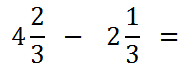Step 1: To change the compound fraction to an improper fraction, we simply multiply the whole number, in this case 4, by the denominator, in this case 3, and then add the numerator, in this case 2. This number is now our new numerator and the denominator remains the same.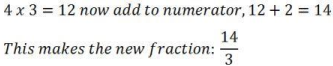We must do this to both fractions in the number sentence.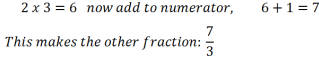Now our new number sentence is: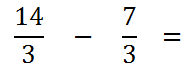Step 2: Denominators are the same

Step 3: Subtract the numerators while keeping the denominator the same. This gives us 7/3, an improper fraction. Change this back to a compound fraction by dividing the numerator by the denominator. Write down the whole number and the remainder becomes the new numerator and the denominator stays the same.

7 divided by 3 gives us 2 with 1 left over so the answer becomes 2 1/3.

Step 4: 2 1/3 is already simplified, so that is our answer.

## Subtracting with Different Denominators

Now let's look at an example where our denominators are not the same:

3 1/4 - 1 2/3

Step 1: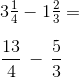To unlock this lesson you must be a Study.com Member.

### Register to view this lesson

Are you a student or a teacher?

#### See for yourself why 30 million people use Study.com

##### Become a Study.com member and start learning now.
Back
What teachers are saying about Study.com

### Earning College Credit

Did you know… We have over 200 college courses that prepare you to earn credit by exam that is accepted by over 1,500 colleges and universities. You can test out of the first two years of college and save thousands off your degree. Anyone can earn credit-by-exam regardless of age or education level.